# Op-Amp Level Shifting Circuit

#### fedezampe

Joined Sep 28, 2020
8
Hi all,

I have an input signal ranging from 0.326V to 3.3V and I need to amplify and shift it so that the output will swing between 0-10V. How can I achieve that using an opamp, please? I know I could do that with a level shifting circuit but I can't figure out how to calculate resistor values.

Any help is much appreciated

Thanks!

#### Ian0

Joined Aug 7, 2020
8,966
This is the circuit that you need: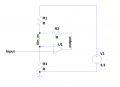Bearing in mind that Vin_m is equal to input. It is just a matter of Kirchhoff's laws and two simultaneous equations.
(Input-Output)/R2+input/R3+(input-3.3)/R1=0 for two cases where Output=10V and input=3.3V and for when Output=0V and input=0.326V.
Hint: the algebra goes more easily if you use conductances instead of resistances and substitute x.G for x/R

•fedezampe

#### crutschow

Joined Mar 14, 2008
33,368
Depending upon your accuracy requirements, you may want to make V1 the same as the op amp supply (as needed to get 10V out from the amp).

#### Papabravo

Joined Feb 24, 2006
20,623
There is a linear transformation, in the form of an equation for straight line, that will do the job. Once you figure that out you can implement in at least two different ways.

#### fedezampe

Joined Sep 28, 2020
8
Hi all,

Thank you very much for your replies.

I have tried the solution that Ian0 proposed and simulated it. It is somewhat working, unfortunately though the output can only reach about 5V. I'm not sure where the problem might lie, I've also tried to increase V1 as advised by crutschow but instead the behaviour became even worse. The calculations, circuit and simulation are attached. Can you show any major issue, please?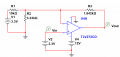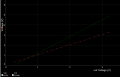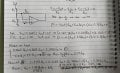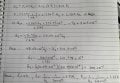This is the circuit that you need:
Bearing in mind that Vin_m is equal to input. It is just a matter of Kirchhoff's laws and two simultaneous equations.
(Input-Output)/R2+input/R3+(input-3.3)/R1=0 for two cases where Output=10V and input=3.3V and for when Output=0V and input=0.326V.
Hint: the algebra goes more easily if you use conductances instead of resistances and substitute x.G for x/R

#### Ian0

Joined Aug 7, 2020
8,966
It usually takes me a few goes to eliminate all the errors!
As a reality check, the gain is equal to 1+(R3/(R1||R2) and that should equal 3.36.

•fedezampe

#### crutschow

Joined Mar 14, 2008
33,368
I don't have the patience to go through your calculations, but below is the simulation using the values I determined:
I try to avoid simultaneous equations, so I calculated the values using the four steps listed.
I hid the values for R2 and R3, but show a starter value for R1 that you can use for the rest of the calculations, if desired.
(I didn't have the op amp model for the one you show, so I used a rail-rail op amp model I have).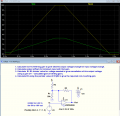Last edited:
•fedezampe

#### fedezampe

Joined Sep 28, 2020
8

#### fedezampe

Joined Sep 28, 2020
8
I don't have the patience to go through your calculations, but below is the simulation using the values I determined:
I try to avoid simultaneous equations, so I calculated the values using the four steps listed.
I hid the values for R2 and R3, but show a starter value for R1 that you can use for the rest of the calculations, if desired.
(I didn't have the op amp model for the one you show, so I used a rail-rail op amp model I have).

View attachment 290685
crutschow, thanks for providing this for me, I have finally managed to get my circuit to work.